Next: About this document ... Up: lab_template Previous: lab_template

Subsections

# Double integrals with Maple

## Purpose

The purpose of this lab is to acquaint you with using Maple to do double integrals.

## Background

### Volumes from double integrals

Suppose that
is a rectangular region in the the
plane, and that
is a continuous, non-negative function on. Then the volume of the solid aboveand below
is given by the double integral
You learned in class that such integrals can be evaluated by either of the iterated integralsor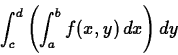where the rectangleis defined by the inequalities
and.

Following are examples of how to use Maple to compute double integrals. It also has an example of how to use Maple if the region of integration is of the more complicated form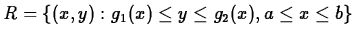. This is the case where the base of the solid is not rectangular, but is bounded by two curves
and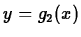. Ifis as before, then the volume of the solid above
and belowis given byThe other case, where the regionis
-simple can also be handled using Maple.

## Maple Commands

The main command for computing multiple integrals with Maple is the int command you are already familiar with. You simply use nested int commands and compute the integrals as iterated integrals. To integrate a function over a rectangular region, just nest the two int commands. The following command computes the integral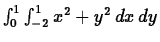>int(int(x^2+y^2,x=-2..1),y=0..1);

This command computes the same integral, but in the opposite order. that is, it computes
>int(int(x^2+y^2,y=0..1),x=-2..1);

Maple can also compute double intgrals where the limits are not constants. For example, suppose you wanted to compute the integral of
over the disk in the x-y plane whose boundary is the circle
. This can be treated as a region that is y-simple by solving the equation of the circle for. This gives two functions
and
, which are just the upper and lower halves of the circle. The integral would be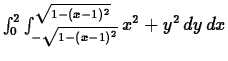and the Maple command to do this integral is
>int(int(x^2+y^2,y=-sqrt(1-(x-1)^2)..sqrt(1-(x-1)^2)),x=0..2);

You can also use Maple to compute double integrals over regions that are x-simple. Suppose we repeat the previous calculation, but solve the equation forinstead of. This gives the two functions
and
for the right and left halves of the circle. The integral would be
and the Maple command to do this is:
>int(int(x^2+y^2,x=1-sqrt(1-y^2)..1+sqrt(1-y^2)),y=-1..1);


## Exercises

1. Use Maple to compute the following double integrals.
a)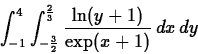b)
c)
2. Letbe the region in the
plane bounded by the two curvesand
. Use a double integral to compute the area of the region. Use both orders of integration. Include a plot of the regionin your worksheet.

3. Use a double integral to find the volume of the region bounded by the two paraboloids
and
.Next: About this document ... Up: lab_template Previous: lab_template
Jane E Bouchard
2013-09-02